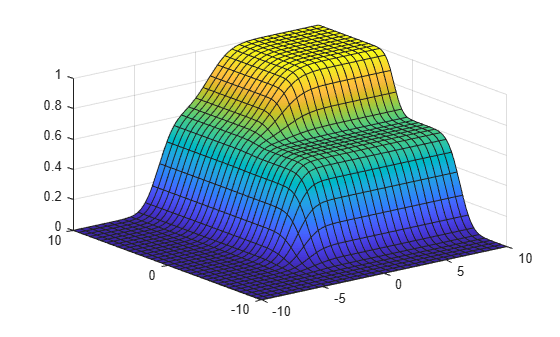# cdf

Cumulative distribution function for Gaussian mixture distribution

## Syntax

``y = cdf(gm,X)``

## Description

example

````y = cdf(gm,X)` returns the cumulative distribution function (cdf) of the Gaussian mixture distribution `gm`, evaluated at the values in `X`.```

## Examples

collapse all

Create a `gmdistribution` object and compute its cdf values.

Define the distribution parameters (means and covariances) of a two-component bivariate Gaussian mixture distribution.

```mu = [1 2;-3 -5]; sigma = [1 1]; % shared diagonal covariance matrix```

Create a `gmdistribution` object by using the `gmdistribution` function. By default, the function creates an equal proportion mixture.

`gm = gmdistribution(mu,sigma)`
```gm = Gaussian mixture distribution with 2 components in 2 dimensions Component 1: Mixing proportion: 0.500000 Mean: 1 2 Component 2: Mixing proportion: 0.500000 Mean: -3 -5 ```

Compute the cdf values of `gm`.

```X = [0 0;1 2;3 3;5 3]; cdf(gm,X)```
```ans = 4×1 0.5011 0.6250 0.9111 0.9207 ```

Create a `gmdistribution` object and plot its cdf.

Define the distribution parameters (means, covariances, and mixing proportions) of two bivariate Gaussian mixture components.

```p = [0.4 0.6]; % Mixing proportions mu = [1 2;-3 -5]; % Means sigma = cat(3,[2 .5],[1 1]) % Covariances 1-by-2-by-2 array```
```sigma = sigma(:,:,1) = 2.0000 0.5000 sigma(:,:,2) = 1 1 ```

The `cat` function concatenates the covariances along the third array dimension. The defined covariance matrices are diagonal matrices. `sigma(1,:,i)` contains the diagonal elements of the covariance matrix of component `i`.

Create a `gmdistribution` object by using the `gmdistribution` function.

`gm = gmdistribution(mu,sigma,p)`
```gm = Gaussian mixture distribution with 2 components in 2 dimensions Component 1: Mixing proportion: 0.400000 Mean: 1 2 Component 2: Mixing proportion: 0.600000 Mean: -3 -5 ```

Plot the cdf of the Gaussian mixture distribution by using `fsurf`.

```gmCDF = @(x,y) arrayfun(@(x0,y0) cdf(gm,[x0 y0]),x,y); fsurf(gmCDF,[-10 10])```## Input Arguments

collapse all

Gaussian mixture distribution, also called Gaussian mixture model (GMM), specified as a `gmdistribution` object.

You can create a `gmdistribution` object using `gmdistribution` or `fitgmdist`. Use the `gmdistribution` function to create a `gmdistribution` object by specifying the distribution parameters. Use the `fitgmdist` function to fit a `gmdistribution` model to data given a fixed number of components.

Values at which to evaluate the cdf, specified as an n-by-m numeric matrix, where n is the number of observations and m is the number of variables in each observation.

Data Types: `single` | `double`

## Output Arguments

collapse all

cdf values of the Gaussian mixture distribution `gm`, evaluated at `X`, returned as an n-by-1 numeric vector, where n is the number of observations in `X`.

## Version History

Introduced in R2007b